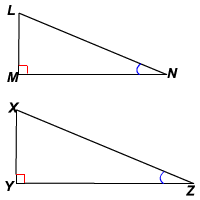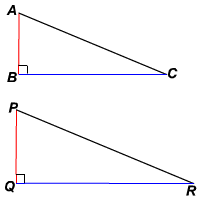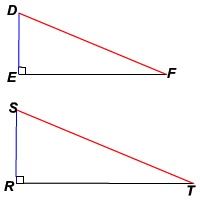# Right Triangle Similarity

Acute Angle Similarity

If one of the acute angles of a right triangle is congruent to an acute angle of another right triangle, then by Angle-Angle Similarity the triangles are similar.In the figure, $\angle M\cong \angle Y$ , since both are right angles, and $\angle N\cong \angle Z$ .

So, $\Delta LMN\sim \Delta XYZ$ .

Leg-Leg Similarity

If the lengths of the corresponding legs of two right triangles are proportional, then by Side-Angle-Side Similarity the triangles are similar.In the figure,  $\frac{AB}{PQ}=\frac{BC}{QR}$ .

So, $\Delta ABC\sim \Delta PQR$ .

Hypotenuse-Leg Similarity

If the lengths of the hypotenuse and a leg of a right triangle are proportional to the corresponding parts of another right triangle, then the triangles are similar. (You can prove this by using the Pythagorean Theorem to show that the third pair of sides is also proportional.)In the figure, $\frac{DF}{ST}=\frac{DE}{SR}$ .

So, $\Delta DEF\sim \Delta SRT$ .

Taking Leg-Leg Similarity and Hypotenus-Leg Similarity together, we can say that if any two sides of a right triangle are proportional to the corresponding sides of another right triangle, then the triangles are similar.